#### Constructing a derived information transition equation

As presented so far, the full history I-state is needed to determine a derived I-state. It may be preferable, however, to discard histories and work entirely in the derived I-space. Without storing the histories on the machine or robot, a derived information transition equation needs to be developed. The important requirement in this case is as follows:

If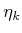is replaced by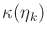, then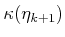must be correctly determined using only,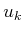, and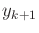.

Whether this requirement can be met depends on the particular I-map. Another way to express the requirement is that ifis given, then the full history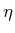does not contain any information that could further constrain. The information provided by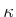is sufficient for determining the next derived I-states. This is similar to the concept of a sufficient statistic, which arises in decision theory . If the requirement is met, thenis called a sufficient I-map. One peculiarity is that the sufficiency is relative to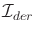, as opposed to being absolute in some sense. For example, any I-map that maps onto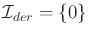is sufficient becauseis always known (it remains fixed at 0). Thus, the requirement for sufficiency depends strongly on the particular derived I-space.

For a sufficient I-map, a derived information transition equation is determined as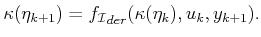(11.27)

The implication is thatis the new I-space in which the problem lives.'' There is no reason for the decision maker to consider histories. This idea is crucial to the success of many planning algorithms. Sections 11.2.2 and 11.2.3 introduce nondeterministic I-spaces and probabilistic I-spaces, which are two of the most important derived I-spaces and are obtained from sufficient I-maps. The I-map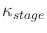from Example 11.12 is also sufficient. The estimation I-map from Example 11.11 is usually not sufficient because some history is needed to provide a better estimate.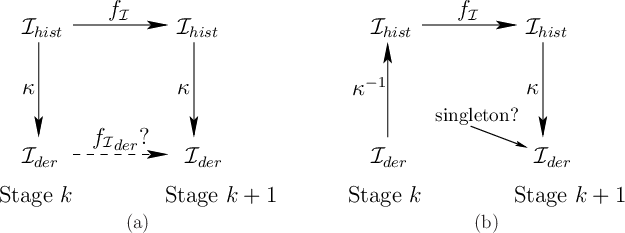The diagram in Figure 11.4a indicates the problem of obtaining a sufficient I-map. The top of the diagram shows the history I-state transitions before the I-map was introduced. The bottom of the diagram shows the attempted derived information transition equation,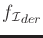. The requirement is that the derived I-state obtained in the lower right must be the same regardless of which path is followed from the upper left. Either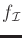can be applied to, followed by, orcan be applied to, followed by some. The problem with the existence ofis thatis usually not invertible. The preimage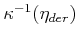of some derived I-state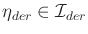yields a set of histories in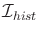. Applyingto all of these yields a set of possible next-stage history I-states. Applyingto these may yield a set of derived I-states because of the ambiguity introduced by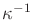. This chain of mappings is shown in Figure 11.4b. If a singleton is obtained under all circumstances, then this yields the required values of. Otherwise, new uncertainty arises about the current derived I-state. This could be handled by defining an information space over the information space, but this nastiness will be avoided here.

Since I-maps can be defined from any derived I-space to another, the concepts presented in this section do not necessarily requireas the starting point. For example, an I-map,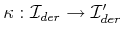, may be called sufficient with respect torather than with respect to.

Steven M LaValle 2020-08-14